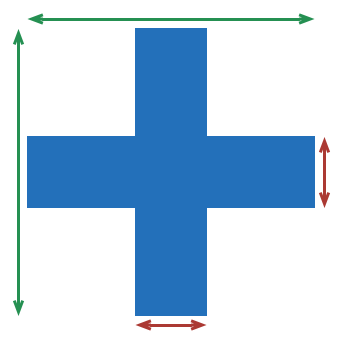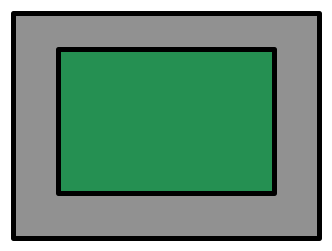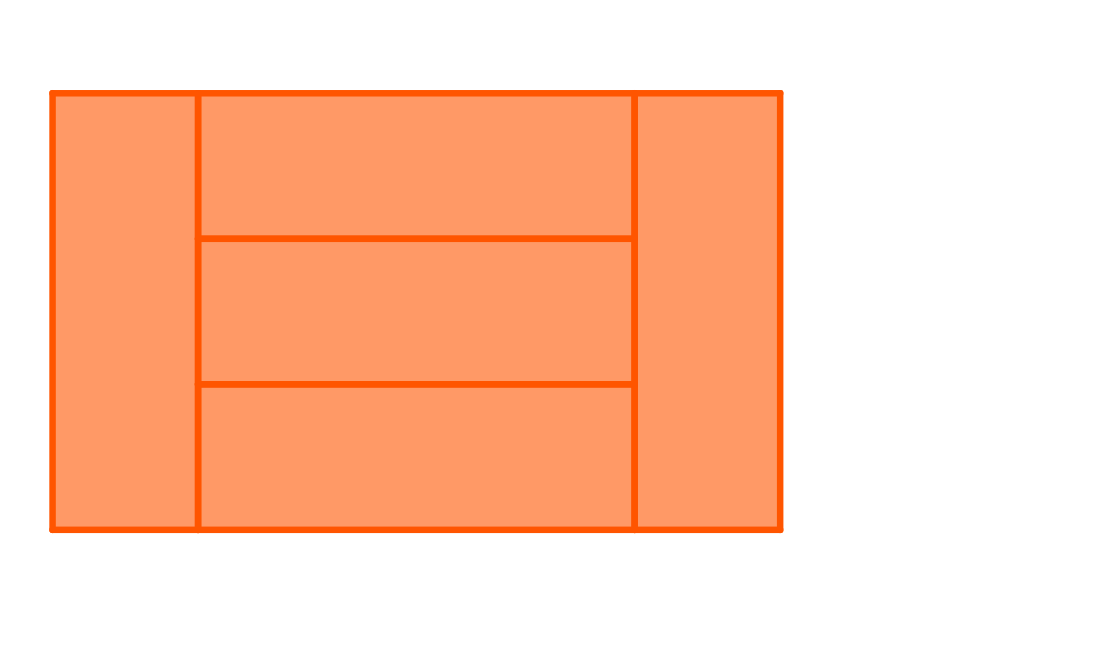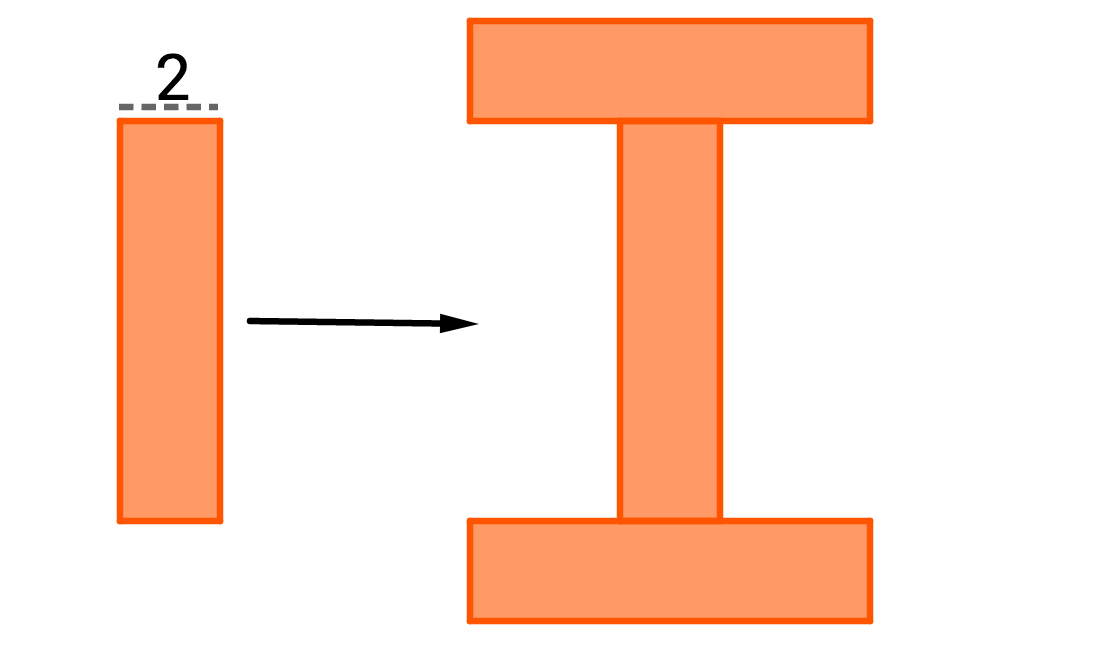Everyday Math

# More Composite FiguresThe above blue cross is formed by two 2 by 7 rectangles. What is its area?There is a garden (the green part of the image) that measures 4 feet by 6 feet. All around it, a path that measures 1 foot wide is constructed. What is the area (in square feet) of the path?In the figure above, a red cross is formed by placing two $7 \times 5$ rectangles over each other. What is the perimeter of the figure?If all of the rectangles are congruent, and the area of the figure is 15, what is the perimeter of the figure?All of the orange rectangles are congruent, and have a width of 2 and perimeter of 20. What is the perimeter of the letter I that is created by placing 3 rectangles side-to-end?

×